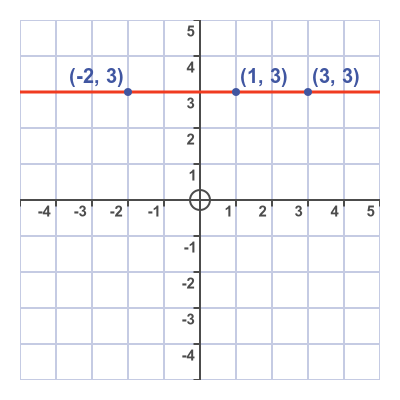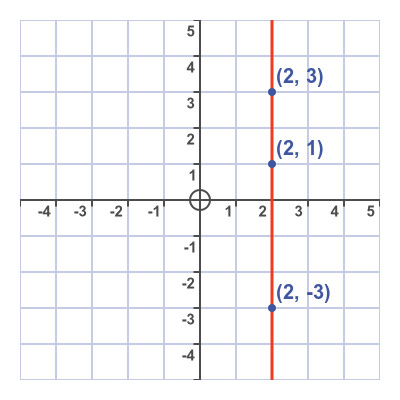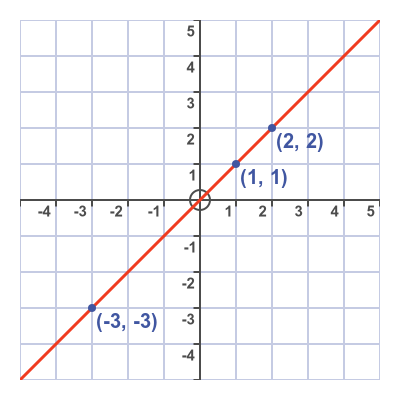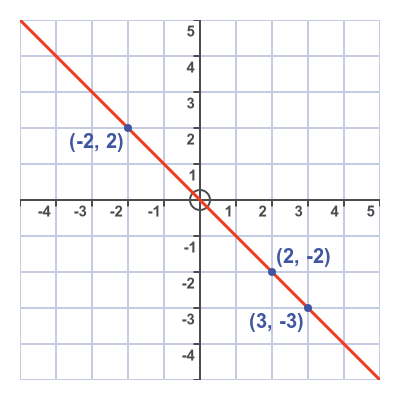# Horizontal, vertical and diagonal straight line graphs

Martin McBride
2022-05-25

In this section, we will look at some simple straight line graphs and their equations.

## Horizontal lines

This graph shows a horizontal straight line:We have marked a few example points that are on the line:

• (-2, 3)
• (1, 3)
• (3, 3)

Each of these points has the same y value of 3, and if you look at the line it is clear that any point on the line will have a y value of 3. So the line has the equation:

$$y = 3$$

This line goes through 3 on the y-axis.

A line that goes through a on the y-axis has the equation:

$$y = a$$

## Vertical lines

This graph shows a vertical straight line:These are points marked on the line:

• (2, -3)
• (2, 1)
• (2, 3)

Each of these points has the same x value of 2, and any point on the line will have an x value of 2. The line has the equation:

$$x = 2$$

This line goes through 2 on the x-axis. A line that goes through a on the x-axis has the equation:

$$x = a$$

Remember that y = a defines all the points with the same y value, so is a horizontal line. x = a defines all the points with the same x value, so is a vertical line.

## Main diagonal lines

Here is the main positive-going diagonal line:This line goes through the origin, in an upwards direction. Here are some of the points the line goes through:

• (-3, -3)
• (1, 1)
• (2, 2)

Every point the line goes through has a y value that is equal to the x value, so the equation is:

$$y = x$$

Here is the main negative-going diagonal line:This line also goes through the origin, this time in a downwards direction. Here are some of the points the line goes through:

• (-2, 2)
• (2, -2)
• (3, -3)

In this graph, every point has a y value that is equal to the negative of the x value, so the equation is:

$$y = -x$$